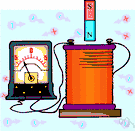# coulomb

(redirected from Coulomb (unit))
Also found in: Thesaurus, Medical, Encyclopedia.

## cou·lomb

(ko͞o′lŏm′, -lōm′)
n. Abbr. C
The basic unit of electric charge, equal to the quantity of charge transferred in one second by a steady current of one ampere, and equivalent to 6.2415 × 1018 elementary charges, where one elementary charge is the charge of a proton or the negative of the charge of an electron. A coulomb's value in the International System differs very slightly from that in the meter-kilogram-second-ampere system of units. See Table at measurement.
Of or relating to the Coulomb force.

[After Charles Augustin de Coulomb.]

## Coulomb

(ˈkuːlɒm; French kulɔ̃)
n
(Biography) Charles Augustin de (ʃarl oɡystɛ̃ də). 1736–1806, French physicist: made many discoveries in the field of electricity and magnetism

## coulomb

(ˈkuːlɒm)
n
(Units) the derived SI unit of electric charge; the quantity of electricity transported in one second by a current of 1 ampere. Symbol: C
[C19: named after Charles Augustin de Coulomb]

## cou•lomb

(ˈku lɒm, -loʊm, kuˈlɒm, -ˈloʊm)

n.
the SI unit of quantity of electricity, equal to the quantity of electric charge transferred in one second across a conductor in which there is a constant current of one ampere. Abbr.: C
[1880–85; after Coulomb]

## Cou•lomb

(ˈku lɒm, -loʊm, kuˈlɒm, -ˈloʊm)

n.
Charles Augustin de, 1736–1806, French physicist and inventor.

## cou·lomb

(ko͞o′lŏm′, ko͞o′lōm′)
A unit used to measure electric charge. One coulomb is equal to the quantity of charge that passes a point in an electric circuit in one second when a current of one ampere is flowing through the circuit.

## coulomb

The unit of electric charge, defined as the quantity of electricity conveyed by one ampere in one second.
ThesaurusAntonymsRelated WordsSynonymsLegend:
 Noun 1coulomb - a unit of electrical charge equal to the amount of charge transferred by a current of 1 ampere in 1 secondcharge unit, quantity unit - a measure of the quantity of electricity (determined by the amount of an electric current and the time for which it flows)abcoulomb - a unit of electrical charge equal to 10 coulombsampere-minute - a unit of charge equal to 60 coulombs 2 Coulomb - French physicist famous for his discoveries in the field of electricity and magnetism; formulated Coulomb's Law (1736-1806)Charles Augustin de Coulomb
Translations
Coulomb

[ˈkuːlɒm] N

## coulomb

nCoulomb nt
Site: Follow: Share:
Open / Close